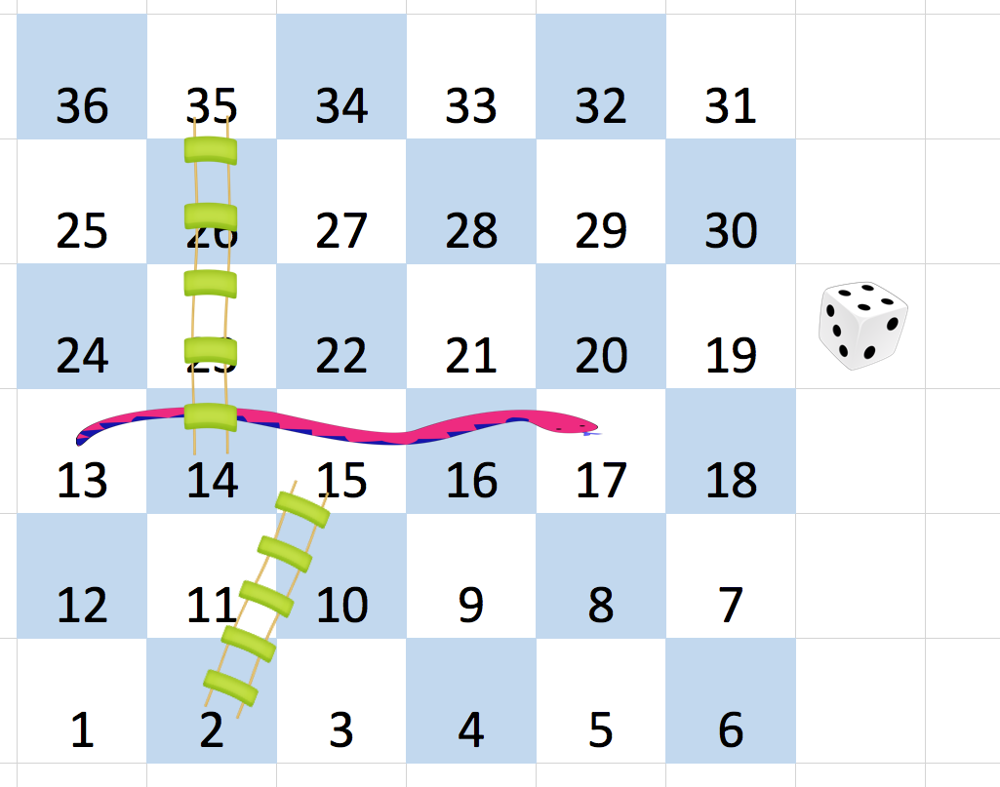# LeetCode: 909. 蛇梯棋¶

## 1、题目描述¶• 你选择一个目标方块 S，它的编号是 x+1，x+2，x+3，x+4，x+5，或者 x+6，只要这个数字 <= N*N
• 如果 S 有一个蛇或梯子，你就移动到那个蛇或梯子的目的地。否则，你会移动到 S。

输入：[
[-1,-1,-1,-1,-1,-1],
[-1,-1,-1,-1,-1,-1],
[-1,-1,-1,-1,-1,-1],
[-1,35,-1,-1,13,-1],
[-1,-1,-1,-1,-1,-1],
[-1,15,-1,-1,-1,-1]]



• 2 <= board.length = board.length <= 20
• board[i][j] 介于 1 和 N*N 之间或者等于 -1。
• 编号为 1 的方格上没有蛇或梯子。
• 编号为 N*N 的方格上没有蛇或梯子。

## 2、解题思路¶

• 使用广度优先搜索
• 需要注意的就是坐标与正常的不同，并且是蛇形排列的
class Solution:
def snakesAndLadders(self, board: List[List[int]]) -> int:
row, col = len(board), len(board)
total = row * col

def get_position(num):
quotient, remainder = divmod(num - 1, col)
pos_row = row - 1 - quotient
pos_col = remainder if quotient % 2 == 0 else col - 1 - remainder
return pos_row, pos_col

visited = {1, }
cur = 
step = 0
while cur:
next_cur = []
for cur_node in cur:
if cur_node == total:
return step
for i in range(cur_node + 1, min(cur_node + 6, total) + 1):
r, c = get_position(i)
n = i
if board[r][c] != -1:
n = board[r][c]
if n not in visited:
next_cur.append(n)